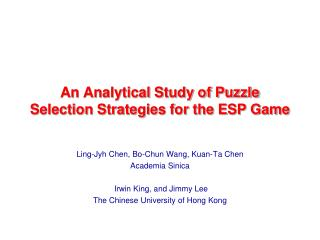DownloadDownload PresentationAn Analytical Study of Puzzle Selection Strategies for the ESP Game

# An Analytical Study of Puzzle Selection Strategies for the ESP Game

Télécharger la présentation## An Analytical Study of Puzzle Selection Strategies for the ESP Game

- - - - - - - - - - - - - - - - - - - - - - - - - - - E N D - - - - - - - - - - - - - - - - - - - - - - - - - - -
##### Presentation Transcript

1. An Analytical Study of Puzzle Selection Strategies for the ESP Game Ling-Jyh Chen, Bo-Chun Wang, Kuan-Ta Chen Academia Sinica Irwin King, and Jimmy Lee The Chinese University of Hong Kong

2. GUESSING: CAR GUESSING: BOY GUESSING: CAR GUESSING: HAT GUESSING: KID SUCCESS! YOU AGREE ON CAR SUCCESS! YOU AGREE ON CAR How the ESP Game Works? PLAYER1 PLAYER 2

3. Why is it Important? • Some statistics (July 2008) • 200,000+ players have contributed 50+ million labels. • Each player plays for a total of 91 minutes. • The throughput is about 233 labels/player/hour (i.e., one label every 15 seconds)

4. The Ideas behind the ESP Game • “Human Computation” represents a new paradigm of applications. • solve some problems which are difficult to be solved by computers • “Games With A Purpose” (GWAP) – by Dr. Luis von Ahn (CMU) • take advantage of people's desire to be entertained • produce useful metadata as a by-product

5. Our Contributions • We propose an evaluation metric for human computation systems. • We study the inner properties of the ESP game using analysis. • We propose an “Optimal Puzzle Selection Algorithm” (OPSA). • We present a comprehensive set of simulation results. • Our model is easy and applicable to other ESP-like systems.

6. The Main Ideas • There are two goals : • the system prefers to maximize the number of puzzles which have been played • the system prefers to take as many labels as possible for each puzzle • Unfortunately, there is a trade-off between the two goals.

7. T : total played rounds r : each puzzle should be played how many times in average the number of the puzzles that have been played the score sum of all produced labels the average scores per puzzle r : each puzzle should be played how many times in average E[S] : the expected score value of each label The Proposed Metric

8. The Proposed Metric

9. Maximum G The Proposed Metric

10. Puzzle Selection Algorithms • Optimal Puzzle Selection Algorithm (OPSA) • select a puzzle based on our analysis • Random Puzzle Selection Algorithm (RPSA) • select a puzzle by random • Fresh-first Puzzle Selection Algorithm (FPSA) • select a puzzle that has been played least frequently

11. Evaluation • Using Monte Carlo Simulations • # of rounds: T = 10,000 • # of puzzles: 100 <= M <= 5,000

12. Conclusion • We have proposed a metric to evaluate the performance of GWAP. • We argue that GWAP needs to be “played with a strategy”. • We propose the Optimal Puzzle Selection Algorithm (OPSA) for ESP-like games.

13. Thank You! http://nrl.iis.sinica.edu.tw